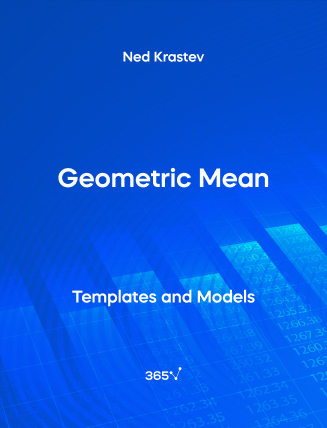## Geometric Mean – Excel Template###### Ned Krastev

Geometric Mean represents the average, or the mean, of a set of values. It is estimated by taking the product of the values we are calculating a geometric mean for.

The uncertainty in cash flows creates a divergence between arithmetic and geometric returns. If there is no variance, arithmetic and geometric returns coincide. In real-world conditions we do have variance so arithmetic returns will be higher than geometric returns, thus representing a more favorable viewpoint.

Other related topics you might be interested to explore are Arithmetic Mean, and Measures of Central Tendency.

This is an open-access Excel template in XLSX format that will be useful for anyone who wants to work as a Statistician, Business Intelligence Analyst, Data Scientist, Portfolio Manager, or Risk Manager.